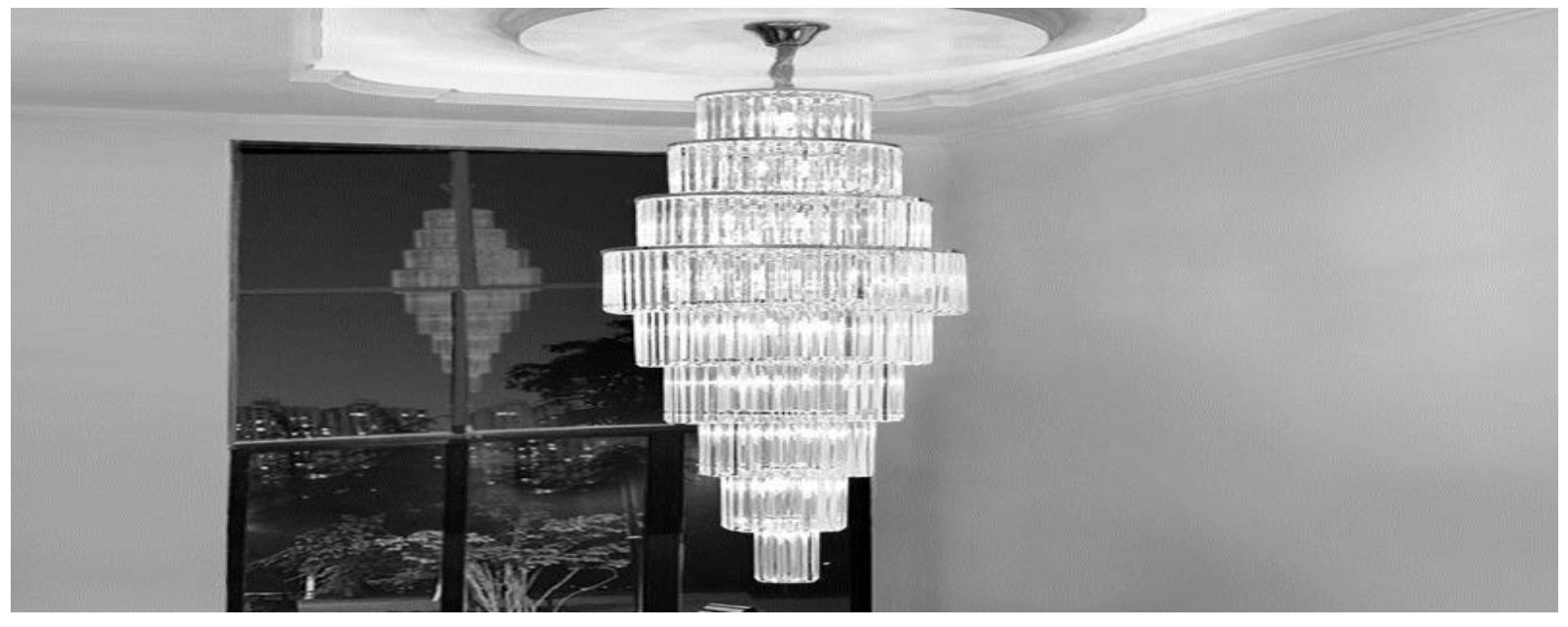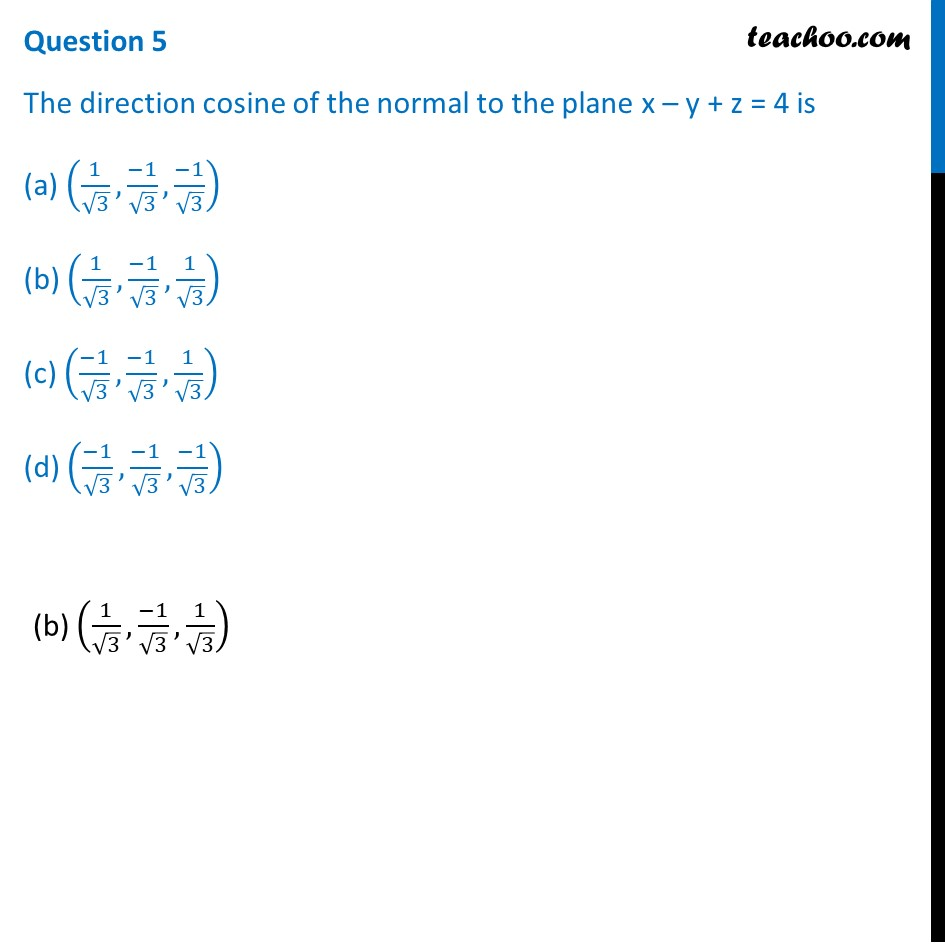## Suppose the floor of a hotel is made up of mirror polished Salvatore stone. There is a large crystal chandelier attached to the ceiling of the hotel room. Consider the floor of the hotel room as a plane having the equation x – y + z = 4 and the crystal chandelier is suspended at the point (1, 0, 1).##1. Chapter 11 Class 12 Three Dimensional Geometry (Term 2)
2. Serial order wise
3. Case Based Questions (MCQ)

Transcript

Question Suppose the floor of a hotel is made up of mirror polished Salvatore stone. There is a large crystal chandelier attached to the ceiling of the hotel room. Consider the floor of the hotel room as a plane having the equation x – y + z = 4 and the crystal chandelier is suspended at the point (1, 0, 1). Based on the above answer the following:Question 1 Find the direction ratios of the perpendicular from the point (1, 0, 1) to the plane x – y + z = 4 (a) (–1, –1, 1) (b) (1, –1, –1) (c) (–1, –1, –1) (d) (1, –1, 1) (d) (1, –1, 1) Question 2 Find the length of the perpendicular from the point (1, 0, 1) to the plane x – y + z = 4. (a) 2/√3 units (b) 4/√3 units (c) 6/√3 units (d) 8/√3 units(a) 2/√3 units Question 3 The equation of the perpendicular from the point (1, 0, 1) to the plane x – y + z = 4 is (a) (𝑥 − 1)/2=(𝑦 + 3)/(−1)=(𝑧 + 5)/3 (b) (𝑥 − 1)/(−2)=(𝑦 + 3)/(−1)=(𝑧 − 5)/2 (c) (𝑥 − 1)/1=𝑦/(−1)=(𝑧 − 1)/1 (d) (𝑥 − 1)/2=𝑦/(−2)=(𝑧 − 1)/1 (c) (𝑥 − 1)/1=𝑦/(−1)=(𝑧 − 1)/1 Question 4 The equation of the plane parallel to the plane x – y + z = 4, which is at a unit distance from the point (1, 0, 1) is (a) 𝑥−𝑦+𝑧+(2−√3) (b) 𝑥−𝑦+𝑧−(2+√3) (c) 𝑥−𝑦+𝑧+(2+√3) (d) Both (a) and (c) (d) Both (a) and (c) Question 5 The direction cosine of the normal to the plane x – y + z = 4 is (a) (1/√3,(−1)/√3,(−1)/√3) (b) (1/√3,(−1)/√3,1/√3) (c) ((−1)/√3,(−1)/√3,1/√3) (d) ((−1)/√3,(−1)/√3,(−1)/√3)(b) (1/√3,(−1)/√3,1/√3)

Case Based Questions (MCQ)